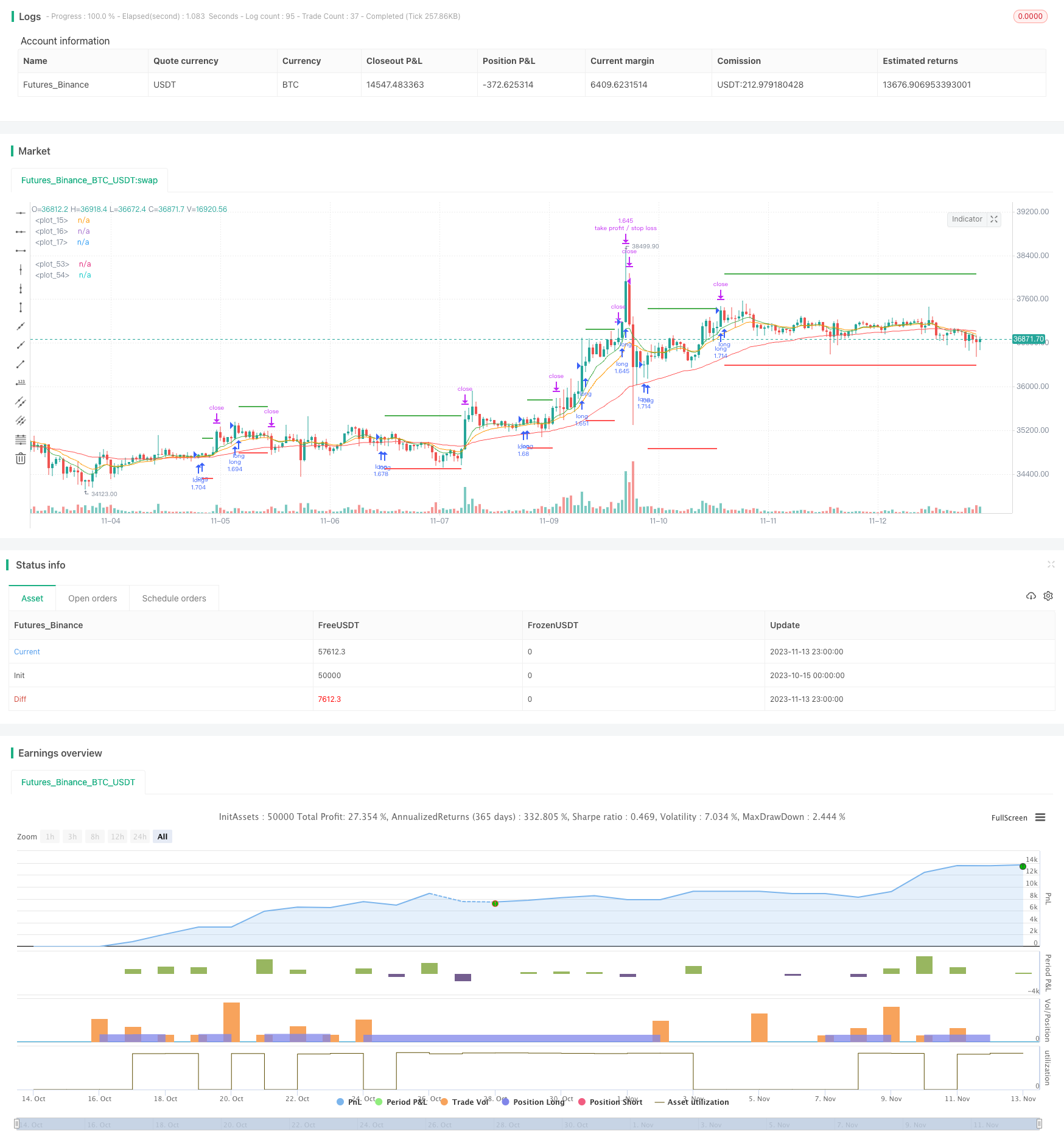# 3EMA with Stochastic RSI Strategy

Author: ChaoZhang, Date: 2023-11-15 10:47:20
Tags:## Overview

This is a trend following strategy combining multiple indicators. It uses three EMAs with different periods, Stochastic RSI and ATR to identify trend direction and establish positions. It goes long when the faster EMA crosses above the slower EMA, with stop loss set at 3 times the recent ATR value and take profit at 2 times the recent ATR.

## Principle

The strategy uses three EMA lines - 8-, 14- and 50-period EMAs, representing price trends over different timeframes. When the 8-period EMA crosses above the 14-period EMA, and the 14-period EMA crosses above the 50-period EMA, it signals the start of an uptrend and a long position can be initiated.

The Stochastic RSI indicator incorporates RSI and Stochastic calculations to identify overbought/oversold conditions. When the Stochastic RSI K line crosses above the D line from below, it suggests the market is transitioning from oversold to bullish outlook, allowing a long position.

ATR represents the recent volatility range. The strategy uses 3 times ATR as stop loss distance and 2 times ATR as take profit distance to lock in profits and control risk.

• EMAs filter out noise in price data and identify trend direction
• Stochastic RSI identifies reversal opportunities
• ATR dynamically trails stop loss/take profit based on market volatility

## Risks

• Multiple indicators may generate conflicting signals
• Fixed stop loss/take profit ratios cannot adapt to changing market conditions
• Short-term longs susceptible to reversals

Optimization can be done by adjusting EMA periods to optimize sensitivity. Making ATR ratios adjustable allows customization based on market conditions. Adding other indicators helps validate signals and avoid mistakes.

## Enhancement

• Adjust EMA periods to optimize sensitivity
• Add other indicators to avoid false signals

## Conclusion

The strategy considers trend, overbought/oversold levels, and volatility range to identify entry timing. EMAs and Stochastic RSI combined effectively identify trends, while ATR’s dynamic stop loss/take profit helps risk control. With parameter tuning and optimizations, the strategy can become a reliable trend following system. But false signals and fixed stop loss/take profit caveats need to be watched out for.

```/*backtest
start: 2023-10-15 00:00:00
end: 2023-11-14 00:00:00
period: 1h
basePeriod: 15m
exchanges: [{"eid":"Futures_Binance","currency":"BTC_USDT"}]
*/

// This source code is subject to the terms of the Mozilla Public License 2.0 at https://mozilla.org/MPL/2.0/

//@version=4
strategy("3 x EMA + Stochastic RSI + ATR", overlay = true, default_qty_type = strategy.percent_of_equity, default_qty_value = 100)

// 3x EMA
ema1Length = input(8, "EMA1 Length", minval = 1)
ema2Length = input(14, "EMA2 Length", minval = 1)
ema3Length = input(50, "EMA3 Length", minval = 1)
ema1 = ema(close, ema1Length)
ema2 = ema(close, ema2Length)
ema3 = ema(close, ema3Length)

plot(ema1, color = color.green)
plot(ema2, color = color.orange)
plot(ema3, color = color.red)

// Stochastic RSI
smoothK = input(3, "K", minval=1)
smoothD = input(3, "D", minval=1)
lengthRSI = input(14, "RSI Length", minval=1)
lengthStoch = input(14, "Stochastic Length", minval=1)
src = input(close, title="RSI Source")
rsi1 = rsi(src, lengthRSI)
k = sma(stoch(rsi1, rsi1, rsi1, lengthStoch), smoothK)
d = sma(k, smoothD)

// ATR
atrPeriod = input(14, "ATR Period")
takeProfitMultiplier= input(2.0, "Take-profit Multiplier")
stopLossMultiplier= input(3.0, "Stop-loss Multiplier")
atrSeries = atr(atrPeriod)

longCondition = ema1 > ema2 and ema2 > ema3 and crossover(k, d)
strategy.entry("long", strategy.long, when = longCondition)

float stopLoss = na
float takeProfit = na

if (strategy.position_size > 0)
if (na(stopLoss))
stopLoss := strategy.position_avg_price - atrSeries * stopLossMultiplier
else
stopLoss := stopLoss
if (na(takeProfit))
takeProfit := strategy.position_avg_price + atrSeries * takeProfitMultiplier
else
takeProfit := takeProfit

strategy.exit("take profit / stop loss", limit = takeProfit, stop = stopLoss)

plot(stopLoss, color = color.red, linewidth = 2, style = plot.style_linebr)
plot(takeProfit, color = color.green, linewidth = 2, style = plot.style_linebr)
```

More